# Category Archives: jFreeChart

## Adding LaTeX equations to Incanter charts

I’ve added a new library to Incanter called incanter.latex that adds the ability to include LaTeX formatted equations as annotations and subtitles in charts. The library is based on the fantastically useful JLaTeXMath library.

The following examples require Incanter version 1.2.2-SNAPSHOT or greater. Add the following dependency to your project.clj file:

[incanter "1.2.2-SNAPSHOT"]


(use '(incanter core stats charts latex))


Define the latex-formatted equation; I’ll use the str function so I can break the equation across multiple lines. Notice that I have to use two backslashes where I would only need one if I were were working directly in LaTeX; this is because the backslash is an escape character in Clojure/Java strings.

(def eq (str "f(x)=\\frac{1}{\\sqrt{2\\pi \\sigma^2}}"
"e^{\\frac{-(x - \\mu)^2}{2 \\sigma^2}}"))


The equation can be rendered as an image with the latex function. The rendered equation can then be viewed in a window or saved as a png file with the view and save functions respectively.

(view (latex eq))
(save (latex eq) filename)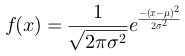Use the add-latex function to add an annotation to a chart. The following example adds the above equation to a function-plot of the Normal PDF.

(doto (function-plot pdf-normal -3 3)
view)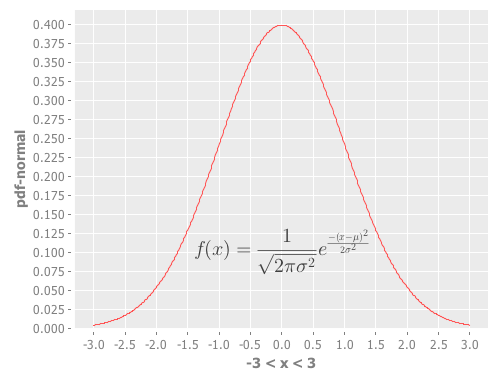Use the add-latex-subtitle function to add a rendered LaTeX equation as a subtitle to the chart (this particular chart does not have a main title).

(doto (function-plot pdf-normal -3 3)
view)The complete code for the above examples can be found here.

## New default theme and customization features for Incanter charts

I just updated Incanter‘s default chart theme. The new theme is inspired by Hadley Wickham‘s awesome ggplot2 package for R.

The first example is my usual “hello world” chart, a histogram of data sampled from a normal distribution.

(use '(incanter core charts stats datasets))

(view (histogram (sample-normal 1000)))The next example is a scatter plot of the sepal-length vs. sepal-width from the built-in iris data set.

(view (scatter-plot :Sepal.Length :Sepal.Width :data (get-dataset :iris)))In addition to changing the default theme, I have included new functions for customizing the appearance of the charts. Here’s an example of the set-stroke function, used here to change the color of the data points in the previous chart.

(doto (scatter-plot :Sepal.Length :Sepal.Width :data (get-dataset :iris))
(set-stroke-color java.awt.Color/gray)
view)The next example uses the :group-by option to color the points based on their species.

(view (scatter-plot :Sepal.Length :Sepal.Width
:group-by :Species
:data (get-dataset :iris)))This example uses function-plot to create an xy-plot of the sine and cosine functions.

(doto (function-plot sin -10 10)
view)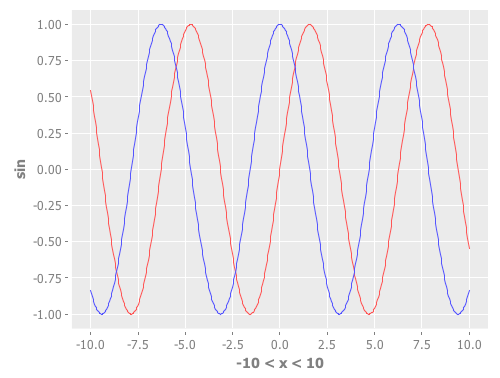This example uses the $rollup and bar-chart functions to plot the data from the built-in hair-eye-color data set. (with-data (->> (get-dataset :hair-eye-color) ($rollup :sum :count [:hair :eye]))
(view (bar-chart :hair :count :group-by :eye :legend true)))This example uses the box-plot function to plot data from three gamma distributions.

(doto (box-plot (sample-gamma 1000 :shape 1 :rate 2)
:legend true :y-label "")
view
(add-box-plot (sample-gamma 1000 :shape 2 :rate 2))
(add-box-plot (sample-gamma 1000 :shape 3 :rate 2)))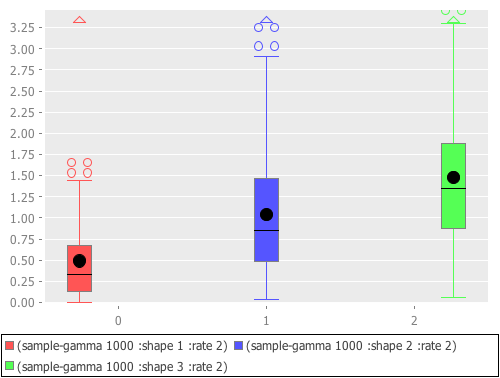The following examples are based on the charts in figure 4.2 of chapter four of “The Joy of Clojure“, where the performance characteristics of Clojure’s data structures are discussed.

First define the two functions to plot, and the range of values to plot them over.

(defn log32 [x] (/ (log x) (log 32)))
(defn f1 [n] (plus (log2 n) (mult (log32 n) 5000)))
(defn f2 [n] n)

(def min-val 10)
(def max-val 40000)


Next, create the plot and use the set-stroke function to increase the stroke thickness for both lines, and make the second line dashed.

(def chart (doto (function-plot f1 min-val max-val
:legend true
:series-label "O(log2 n) + O(log32 n) * 5000"
:x-label ""
:y-label "")
:step-size 5000
:series-label "O(n)")
(set-stroke :width 2)
(set-stroke :width 2 :dataset 1 :dash 5)))

(view chart)


The three charts in the book are of the same data but each focuses on a different region. You can use the set-y-range and set-x-range functions to zoom-in on each of the different regions.

;; PLOT (A)
(doto chart
(set-title "(A)")
(set-x-range 100 5000)
(set-y-range 30 12000));; PLOT (B)
(doto chart
(set-title "(B)")
(set-y-range 10000 16000)
(set-x-range 10000 16000));; PLOT (C)
(doto chart
(set-title "(C)")
(set-y-range 0 30000)
(set-x-range 0 30000))The new theme is available in the latest version of Incanter on Clojars and Github, and the complete code for the above examples is available here.

## Dynamic charts with Incanter

I had the opportunity to attend last week’s PragmaticStudio Clojure workshop taught by Rich Hickey and Stuart Halloway (I highly recommend it, and there are still seats open for the May class). During the three days I talked with Rich about features he’d like to see in Incanter, and the first thing he asked about was adding dynamic charts, like are available in Mathematica using the Manipulate function. So I ended up spending much of my lab time working on this feature, the first draft of which is now available.

Incanter has three new macros, sliders, dynamic-xy-plot, and dynamic-scatter-plot. The sliders macro can bind multiple named sequences to an equal number of JSlider widgets. When a slider is manipulated a user defined expression is evaluated. For instance, the following code will display two slider widgets bound to two sequences, x and y.

(sliders [x (range -3 3 0.01)
y (range 0.01 10 0.1)]
(foo x y))


Each time one of the sliders is manipulated the expression (foo x y) will be evaluated with the new value of either x or y. Presumably, foo has side effects, like changing the value of a ref or manipulating a GUI widget, since it is running in the separate thread used by the slider widget.

I then combined this macro with incanter.charts/set-data function to create dynamic versions of xy-plot and scatter-plot, named appropriately dynamic-xy-plot and dynamic-scatter-plot respectively.

The following example creates an xy-plot of a sequence of values named x versus the normal PDF of x, and displays two sliders bound to the mean and standard deviation of the PDF.

(let [x (range -3 3 0.1)]
(view (dynamic-xy-plot [mean (range -3 3 0.1)
std-dev (range 0.1 10 0.1)]
[x (pdf-normal x :mean mean :sd std-dev)])))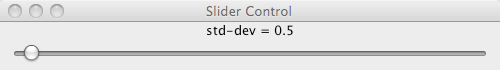The expression provided to dynamic-xy-plot must produce a sequence containing either two sequences with N values, or N sequences with two values each. In other words, a N-by-2 matrix or a 2-by-N matrix, where N is the number of points plotted. The expression above,

[x (pdf-normal x :mean mean :sd std-dev)]

produces a vector containing a sequence called x and the sequence produced by calling pdf-normal on x (equivalent to a N-by-2 matrix).

Manipulating the sliders will change the shape and position of the curve.The dynamic-scatter-plot macro works the same way as dynamic-xy-plot. All three macros are available in the version of Incanter on Github and in repo.incanter.org.

## Data Sorcery with Clojure & Incanter: Introduction to Datasets & Charts

I put together my slides (pdf) for next week’s National Capital Area Clojure Users Group February Meetup. Being snow-bound this week, I’ve been able to make more slides than I’ll have time to cover during next week’s session, so I’ll be skimming over some of the examples.

Russ Olsen will start the session with an introduction to Clojure, so if you’re in the D.C. area next Thursday (February 18), sign-up for the meetup.The code used in this presentation is available here, and a more printer-friendly version of the presentation itself, with a white background, is available here.

## Dark theme for Incanter charts

JFreeChart has been a fantastic library, I’ve been able to include useful charting functionality in Incanter very quickly because of it, but I’m not a big fan of its default visual theme. Eventually I’d like to create some new themes, or better yet include themes created by others, but in the meantime I have created the set-theme function, which accepts a chart and either a keyword indicating a built-in theme or a JFreeChart ChartTheme object, and applies the theme to the chart.

At the moment, the only built-in themes are :default and :dark, but hopefully that will change in the future.

Here’s an example of using set-theme. First I’ll create a chart with the default theme,

(use '(incanter core charts datasets))

(with-data (get-dataset :iris)
(view (scatter-plot :Sepal.Length :Sepal.Width :group-by :Species)))and here’s the same scatter-plot with the dark theme.

(with-data (get-dataset :iris)
(doto (scatter-plot :Sepal.Length :Sepal.Width :group-by :Species)
(set-theme :dark)
view))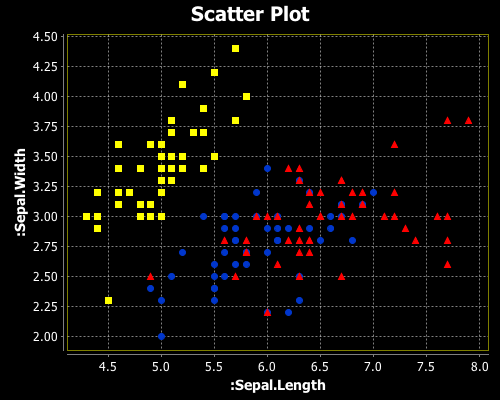The set-theme function is available in the latest version Incanter @ Github.

I have also added the incanter-pdf module discussed in the previous blog post, but it isn’t installed by default. To install it in your local Maven repository, run ‘mvn install’ from the incanter/modules/incanter-pdf directory.

## Saving Incanter charts as PDF documents

Incanter charts can be saved as PNG files using the save function, but I had a request earlier today to add the ability to save them as PDF documents.

So I’ve created a new function called save-pdf in a new package called incanter.pdf.

Here’s a basic example.

(use '(incanter core charts pdf))
(save-pdf (function-plot sin -4 4) "./pdf-chart.pdf")

Which outputs the following PDF file.